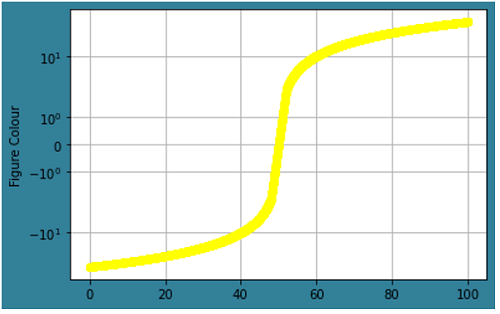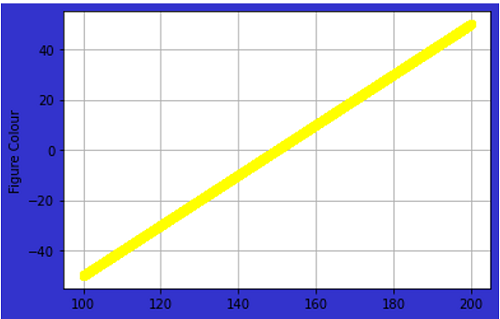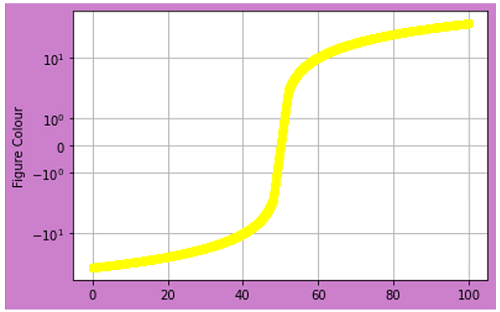# Python | Background Color of the Plot

In this article, we are going to set background color in a matplotlib figure (plot)?
Submitted by Anuj Singh, on August 12, 2020

In some cases, we can need a different background color, and therefore, in this article, we are going to convert the default white background to a different color.## Python code for background color of the plot

```import matplotlib.pyplot as plt
import numpy as np

dt = 0.02
x = np.arange(-50.0, 50.0, dt)
y = np.arange(0, 100.0, dt)

plt.figure(facecolor=(0.2,0.5,0.6))
plt.plot(y, x, 'o', color='yellow')
plt.yscale('symlog')
plt.ylabel('Figure Colour')
plt.grid()

plt.figure(facecolor=(0.2,0.2,0.8))
plt.plot(y+100, x, 'o', color='yellow')
plt.ylabel('Figure Colour')
plt.grid()
plt.show()

plt.figure(facecolor=(0.8,0.5,0.8))
plt.plot(y, x, 'o', color='yellow')
plt.yscale('symlog')
plt.ylabel('Figure Colour')
plt.grid()
```

Output:

```Output is as Figure
```